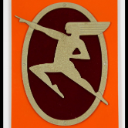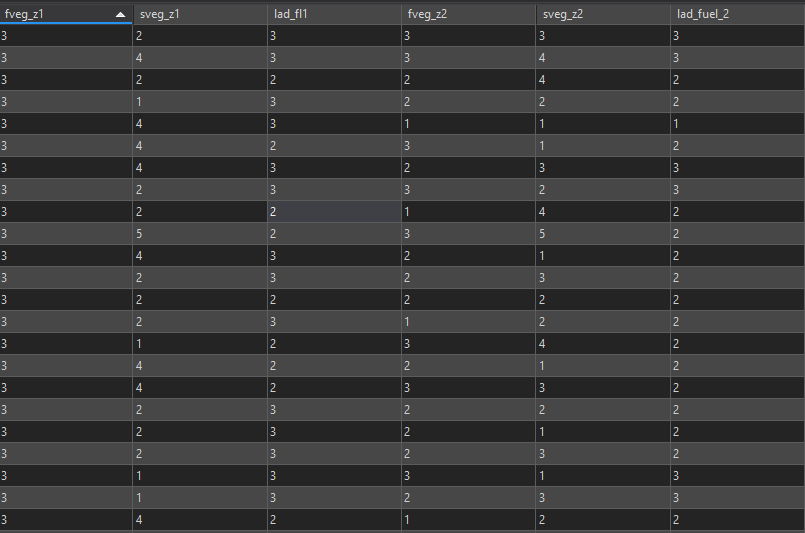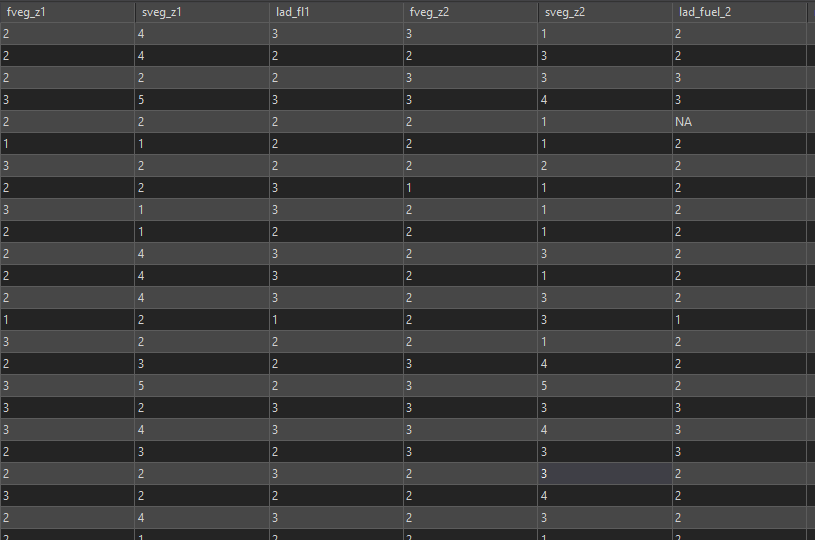# IF AND OR Statement - Arcade

1785
11
08-04-2020 03:21 PMbyMVP Regular Contributor

I can write what I am trying to do in excel but not in Arcade.

I am looking for a way to write something like this:

I have an IF statement followed by an AND with a bunch of OR statements.

 =IF(AND(OR(K4=1,K4=4),OR(L4=2,L4=1),OR(M4=2,M4=1),OR(N4=1,N4=4),OR(O4=2,O4=1),OR(P4=2,P4=1)),"0",IF(AND(OR(K4=2,K4=3,K4=5),L4=3,M4=3),"250","150"))'
Tags (3)
1 Solution

Accepted SolutionsbyMVP Esteemed Contributor

I tried this in the Arcade playground and it returns 0. Changing the three numbers as commented will return 250, but changing only one number will return 150

``````var fveg_z1=1; //5 These three values yields 250
var sveg_z1=2; //3 These three values yields 250
var lad_fl1=2; //3 These three values yields 250
var fveg_z2=1;
var sveg_z2=2;

var result = IIf((fveg_z1==1 || fveg_z1==4) &&
(sveg_z1==2 || sveg_z1==1) &&
(fveg_z2==1 || fveg_z2==4) &&
(sveg_z2==2 || sveg_z2==1) &&
IIf((fveg_z1==2 || fveg_z1==3 || fveg_z1==5) &&
sveg_z1==3 &&
"150")
);
return result;‍‍‍‍‍‍‍‍‍‍‍‍‍‍‍‍‍‍‍``````

What are the actual values for those fields?

11 RepliesbyEsri Esteemed Contributor

Hi dafiter ,

See below a translation of the Excel formula into Arcade and the first lines to specify values for testing:

``````var k = 2; // 1-5
var l = 3; // 1-3
var m = 3; // 1-3
var n = 1; // 1,4
var o = 1; // 1,2
var p = 1; // 1,2

var result = IIf((K==1 || K==4) && (L==2 || L==1) && (M==2 || M==1) && (N==1 || N==4) && (O==2 || O==1) && (P==2 || P==1), "0", IIf((K==2 || K==3 || K==5) && L==3 && M==3, "250","150"));
return result;‍‍‍‍‍‍‍‍‍``````byMVP Regular Contributor

Thank you for helping me with this.

Here is the code after I plug in my fields.

``````var result = IIf((\$feature.fveg_z1==1 || \$feature.fveg_z1==4) && (\$feature.sveg_z1==2 || \$feature.sveg_z1==1) && (\$feature.lad_fl1==2 || \$feature.lad_fl1==1) && (\$feature.fveg_z2==1 || \$feature.fveg_z2==4) && (\$feature.sveg_z2==2 || \$feature.sveg_z2==1) && (\$feature.lad_fuel_2==2 || \$feature.lad_fuel_2==1), "0", IIf((\$feature.fveg_z1==2 || \$feature.fveg_z1==3 || \$feature.fveg_z1==5) && \$feature.sveg_z1==3 && \$feature.lad_fl1==3, "250","150"));
return result;‍‍``````

All I get for answers is 150. I know that some should be 0 or 250.

Did I plug it in wrong?byMVP Esteemed Contributor

I tried this in the Arcade playground and it returns 0. Changing the three numbers as commented will return 250, but changing only one number will return 150

``````var fveg_z1=1; //5 These three values yields 250
var sveg_z1=2; //3 These three values yields 250
var lad_fl1=2; //3 These three values yields 250
var fveg_z2=1;
var sveg_z2=2;

var result = IIf((fveg_z1==1 || fveg_z1==4) &&
(sveg_z1==2 || sveg_z1==1) &&
(fveg_z2==1 || fveg_z2==4) &&
(sveg_z2==2 || sveg_z2==1) &&
IIf((fveg_z1==2 || fveg_z1==3 || fveg_z1==5) &&
sveg_z1==3 &&
"150")
);
return result;‍‍‍‍‍‍‍‍‍‍‍‍‍‍‍‍‍‍‍``````

What are the actual values for those fields?byMVP Regular Contributor

Here is a sample of the databyMVP Esteemed Contributor

Since for this subset has fveg_z1=3, you're only going to get either 250 or 150. But none of the sveg_z1 value is 3, so all of these records will return 150byMVP Regular Contributor

Sorry that was just a screen shot. There are over 6000 records with all combinations in each field.byMVP Esteemed Contributor

Have you run a selection or filter on the data to verify the different combinations to get 0 or 250?byMVP Regular Contributor

I have only ran it against the whole database. I will try a small subset and see what I get

Update:

I just ran against what I would expect only 0 scores and I still get 150byMVP Regular Contributor

Does it matter if the fields sveg_z1 etc are text not long?